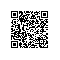# 方差，标准差，协方差、期望值

有一组数据{4,5,6,7}, 平均值为：(4+5+6+7)/4=22/4=5.5

其方差为：[(4-5.5)2+(5-5.5)2+(6-5.5)2+(7-5.5)2]/4

例如上面那组数据的标准差为：{[(4-5.5)2+(5-5.5)2+(6-5.5)2+(7-5.5)2]/4}0.5

概率论统计学中，协方差用于衡量两个变量的总体误差。而方差是协方差的一种特殊情况，即当两个变量是相同的情况。

期望值分别为E(x) = μ 与 E(y) = ν 的两个实数随机变量xy之间的协方差定义为：

其中，E是期望值。它也可以表示为：

直观上来看，协方差表示的是两个变量总体的误差，这与只表示一个变量误差的方差不同。

其中E(x)的计算方法例如：

有两组数据X和Y，{X1=3,X2=4,X3=8},{Y1=2,Y2=5,Y3=5}

E(XY)=(3*2+4*5+8*5)/3=66/3=22

件不确定的事件有确定的所有结果，把第一种的结果值记为s1，它发生的概率记为p1，第二种结果值记为s2，它发生的概率为p2，... 第n种结果值记为sn，它发生的概率记为pn ... 那么期望值 Ex=s1*p1+s2*p2+...+sn*pn+...使用钉钉扫一扫加入圈子
+ 订阅